Home

# Particle Physics

For the longest time as history records, science has held that all matter is composed of fundamental building blocks. Even though they could not see it, the ancient Greeks for example presumed that a stone could be ground up into finer and finer grains until it reached single indivisible points of matter which they called átomos, meaning “uncuttable”. Their suspicions proved correct, as some two-and-a-half-thousand years later scientists in the early 20th century discovered that indivisible unit and named it the atom.

This naming turned out to be premature as it was later found these atoms could be further broken into smaller particles, namely the proton, neutron and electron. But that was not the end of the tale. Over the following decades particle accelerator experiments revealed there to be large number of, what were labeled, sub-atomic particles. This gave birth to a new branch of science called particle physics.

#### Developmental Problems

As time passed and more and more particles were discovered, it became clear that something was amiss with these ‘fundamental’ units of nature. Their numbers ran into tens then to over a hundred. Could nature be this complicated? A study of their properties and interactions led to the idea that many of these were made up of still smaller units. This led to the discovery of quarks, which are said to compose protons, neutrons and other particles.

While it is true that a large number of particles might pose a philosophical problem, a more fundamental problem must be the way in which they are said to interact. In the world of particle physics, matter is constantly flashing in and out of existence as new particles are created and destroyed. And while this process may seem strange, it is stranger still that many of these interactions appear to occur without regard to mass conservation. Take muons for example.

#### Muons

Muons are charged particles that are primarily generated as a result of cosmic bombardment in the upper atmosphere. They are mostly negatively charged and can be thought of as heavy but unstable electrons. Muons have a short half-life of 2.2 microseconds, after which they decay into an electron and a couple of neutrinos. The decay process goes like this: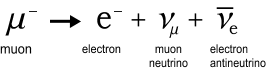This reaction obeys the charge conservation rule in that both muon and electron have an equal negative charge while the neutrinos are neutral. But a muon is 206 times heavier than an electron and the neutrinos weigh nothing (or next to nothing). Where did all that mass go? According to modern physics, mass must either be conserved or converted to an equivalent amount of energy, determined via the E=mc2 relation. This energy must be released in the form of electromagnetic radiation, i.e. as photons. But there is no suggestion in the standard texts that photons are released during this process.

Actually, the above diagram is incomplete because there should also be a W boson particle involved. This W particle weighs in at 157 thousand times heavier than an electron and quickly flashes in-and-out of existence while creating the electron and one of the neutrinos. Here again is another apparent violation of mass conservation, and a huge one at that! But since it quickly disappears we could give it the benefit of doubt and say that it causes no overall conservation problem.

One possibility for mass conservation may have to do with neutrino momentum. I will discuss this further on.

#### Pions

The next question has to do with where muons come from. Muons come from pion decay, which in turn are generated from high-energy proton collisions in the upper atmosphere. The pion to muon conversion process looks like this: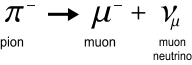Again there is a temporary intermediate W particle involved which I’ve not shown. The pion has a mass of 273 electrons which is only slightly above the muon (at 206) and there are no photons in sight. Hence again we have a mass conservation problem, albeit only minor. Ignoring the various neutrinos then, the complete process goes something like this: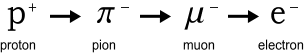Notice something amiss? That’s right: the positive proton yields a negative pion! This is surely impossible according to charge conservation rules. Now to be fair, the interaction is not stated in full like this. Various literatures on the subject discuss the pion/muon and muon/electron decays separately and each decay process shown preserves charge correctly. But when it comes to the full process the literature becomes somewhat vague, particularly in regard to the pion’s charge. For example on Wikipedia’s muon page we find the following :

When a cosmic ray proton impacts atomic nuclei of air atoms in the upper atmosphere, pions are created. These decay within a relatively short distance (meters) into muons (the pion's preferred decay product), and neutrinos.

The above excerpt does not say what charge these pions have except they are somehow created from protons. Since protons are positive this indicates the created pions must also be positive, in which case they could not decay into negative muons. The webpage from SLAC helps clear this up when it says :

In cosmic ray showers, both muons and antimuons are produced about equally.

That’s good. With equal amounts of muons (negative) and antimuons (positive), charge is conserved. But where are all these antimuons? If they are produced in equal numbers and have equal half-lives, we should observe them equally at sea level. Instead the literature indicates a vast abundance of muons.

#### An explanation of pion/muon conversions

The above raises many questions. Does the cosmic proton convert directly to pions or does it create the pions as part of a collision, while preserving its own existence? And what happened to all that mass? Did the mighty cosmic proton convert itself to a puny electron without releasing the required amount of radiation to account for mass difference?

Given the uncertainty over the charge conservation problem in pion creation, it is understandable that the available literature is somewhat vague on details. However I believe there is a better explanation for the above reactions that not only preserves charge and mass, but also does away with these mysterious particle disappearance and creation-out-of-nothing conjectures.

Start with the proton. As was discussed in the chapter on nuclear forces, a proton is believed to be made up of two up-quarks (positive) and one down-quark (negative). Is it possible that these quarks are really the pions and muons we observe? I.e., is the negative muon really a down-quark and the positive pion an up-quark?

According to scientists, protons are essentially unbreakable and quarks can never be seen on their own. But this seems unlikely. If a proton is made of several parts, and you hit it hard enough, e.g. in a high-energy cosmic collision, then surely those parts would separate. Scientists have declared that quarks have fractional charges and, given that we’ve never seen a fractional charge, we’ve obviously never seen a broken proton. This idea of fractional charges was initially introduced to explain the composition of the neutron purely in terms of quarks. But as pointed out in the previous chapter, neutrons can be more easily explained in terms of a proton joined to an electron. Could it be that quarks in fact have unit charges and have been hiding in plain sight all along?

With this idea in mind, let’s assume our proton has just smashed into some part of the atmosphere, e.g. a nitrogen nucleus, and has split into three quarks: two positive and one negative. What now? Refer to the chapter on matter-energy conversion. There it was postulated than an electron and positron can overlap, creating an effectively invisible composite particle, which I called a poseltron. Could a similar event be happening here? The positive quarks are surrounded by electrons. They could quickly absorb one each and become an ‘invisible’ neutral particle. The pions have apparently decayed!

But the down-quark is negative and cannot absorb electrons so it continues down. This is the muon we see. What will happen to it – will it absorb a positron and also disappear? Perhaps, but this is unlikely because there are few positrons to be had; they’ve already been absorbed by available electrons and become poseltrons. Here’s instead what happens. The high-velocity muon collides with a poseltron. This causes the electron-positron pair to split. The positron is absorbed into the muon and the electron is ejected. Voila! The muon ‘decayed’ into an electron. Of course the muon is still there but as it’s now overlapping a positron, it forms a particle of neutral charge and can no longer be seen.

Here’s the full process in picture form: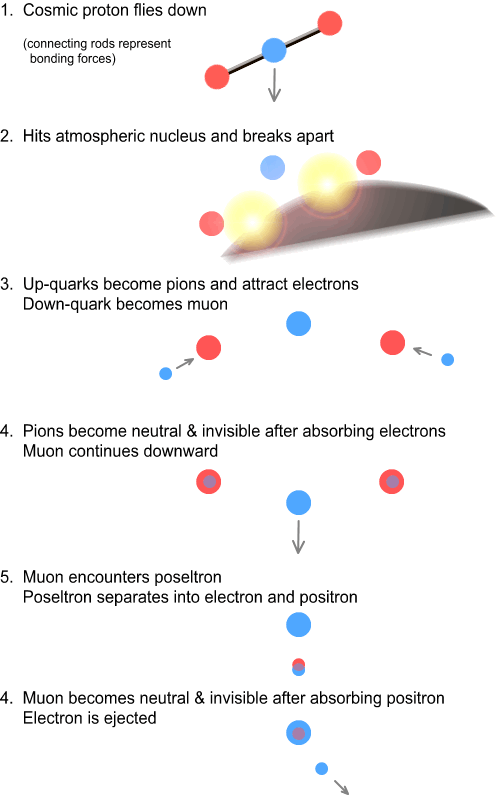As can be seen, no fundamental particles have been created or destroyed, while charge and mass are conserved throughout.

#### Some points on pion/muon lifespan and mass

The pion has a much shorter lifespan than a muon: about 85 times shorter (0.026 vs. 2.2 microseconds). Based on the above, that’s to be expected. Electrons are everywhere and will quickly be absorbed by a pion. But poseltrons and positrons are rare. Hence a pion will only last a few hundred metres before being absorbed, whereas a muon can often make it all the way to sea level.

If a muon is really a down quark this means a down-quark weighs around 206 electron masses, i.e. about one ninth of a proton. Since there are also two up-quarks in a proton this means that an up-quark should weigh (1836-206)/2 = 815 electron masses. There’s a problem here because a pion reportedly weighs only 273 electron masses. So either I’m way out on the assumptions or there’s something fishy about the way a pion’s mass is measured.

#### Neutrinos

Now some discussion needs to be made about neutrinos because I’ve ignored them in the above interactions. Neutrinos are neutral particles emitted during certain decay processes such as neutron decay and the pion and muon decays described above. They have a mass of zero or, according to recent speculations, very close to zero. The existence of neutrinos was first postulated by Wolfgang Pauli in 1930 to explain why electrons leaving a nucleus in the form of beta radiation move more slowly than expected. They were later observed/confirmed in bubble chamber experiments.

That a neutral particle could be observed however comes as a startling claim. By any interpretation of Coulomb’s or Maxwell’s laws, a purely neutral particle (i.e. containing no charges) could not apply any force on a charged particle, nor could it be affected by a static or electromagnetic field. An answer to this may be that they can be observed when impacting another particle directly. The below image shows the bubble chamber experiment in which a neutrino was first detected .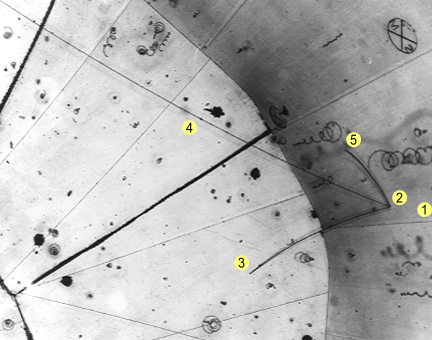The accepted interpretation of this is: (1) a neutrino came in from the right (it can’t be seen due to having no charge). (2) It hits a proton. (3) A positive pion is produced and curves downward. (4) A negative lighter muon is also produced and moves quickly to the left, curving weakly upward. (5) The original heavier proton survives; it moves slowly and curves downward.

According to the standard particle physics model, when oppositely charged particles meet they should annihilate. Apparently the rules are different for neutral particles; namely that they should bounce off other particles like billiard balls, and this requires sub-atomic particles be slightly elastic. Assuming this is true, how can we calculate the degree of elastic bounce? In any classical situation this would be easily solved in terms of momentum and energy conservation. Knowing the momentum requires knowing the mass and this is a problem because neutrinos are commonly assumed to have none.

Special relativity tells us that particles having zero mass, such as photons, must travel at light speed. This is due to the relativistic mass formula, which says an object’s mass increases toward infinity as it nears light speed. For an object of non-zero mass this puts the brakes on acceleration and keeps v below c. But for an object with zero mass the acceleration can only stop when the particle hits light speed, at which point the object gains a non-zero relativistic mass. What will this mass be? To determine this we can use the Lorentz Transform equation: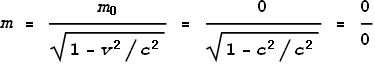Where m0 is the rest mass.

In mathematical terms, zero divided by zero is called an indeterminate, meaning that it can have any real-number value, or even an infinite one. Calculating momentum requires multiplying this indeterminate mass by velocity, in this case c, which of course just gives us another indeterminate. This is not helpful!

But could a neutrino with indeterminate mass/momentum account for the mysteries it is said to solve, such as muon/electron mass-loss and the collision in the above image? After all, if it’s indeterminate then we can assign any value we want to it, right? Perhaps, but we’d be hard-pressed to explain why identical objects moving at the same velocity have different relativistic masses. After all, the speed of light is a universal constant; not a universal variable.

So if neutrinos don’t account for the above collision what does? Here’s my interpretation. The invisible particle coming from the right (1) is actually a positive pion and negative muon overlapping (similar to the poseltron concept). It strikes the proton (2) and this causes the pion and muon to break apart and become visible. The three particles, pion, muon and proton (3,4,5 respectively), are then scattered.

A far more interesting aspect of this image arises from measuring the extent of scattering. A simple pixel measurement shows the length of each track to be:

 Proton 72 Pion 155 Muon >442

This scatter distance represents velocity which, due to momentum conservation, should be inversely proportional to mass. From this we see the pion has approximately half the mass of the proton. A ratio of the pion/proton track lengths gives 72/155 = 0.465. Now see the paragraph farther up where I speculated that an up-quark weighs 815 electron masses. Taking the ratio of up-quark to proton mass we get 815/1836 = 0.444. That’s very close to the pion/proton ratio! Hence it’s quite likely that a pion is an up-quark after all.

In the muon’s case, it ended up off screen so I can only calculate a ratio of 442/72 = 6.1, which is understandably less than the real muon/proton ratio (about 9) because the track must be longer. But seeing as the muon’s mass has already been determined in other experiments by comparing its particle tracks to electrons, we can accept their stated mass as being 206 electrons.

#### Neutral composite particles

This description of a pion-muon particle (which struck the proton in the above image) as well as the poseltron spoken about in the earlier chapter gives rise to the possibility that there are many neutral composite particles in existence. Here are some charged particles we commonly know of:

 Negative Positive Electron Positron Muon Antimuon Pion- Pion+

Looking at this table we see there are nine ways that positive and negative charges could combine to make a neutral particle. The electron-positron pair has been discussed already and it is worth considering the evidence for other combinations. One possible combination is the ‘neutral pion’.

The neutral pion (symbol pi0) is a type of pion having no charge. It has a very short half life of 8.4x10-17 seconds and decays into two photons. According to the literature, this pion is made of four quarks: up, down, anti-up and anti-down. By comparison the charged pions are made of only two quarks each. Despite having twice as many quarks, the neutral pion is somehow lighter than a charged pion: 264 versus 273 electron masses. Again we have another mass conservation problem, and this indicates the assumed particle composition is incorrect.

Here is another interpretation of its decay process. The neutral pion is made of two opposite charges particles. The electric field from a passing photon temporarily forces them apart. They quickly come back together. In the process they oscillate through each other and create electromagnetic waves (photons) similar to the poseltron oscillation process. If we know the frequency of these photons this would give a clue as to the particle’s masses and allow us to determine which particles were involved. Based on the abundance of particle types, the most likely candidate would be a positive pion and negative muon, i.e., an up/down-quark combination.

#### A Bold Hypothesis

In the above description of muon to electron conversion, the muon decayed when it met a poseltron. This caused the electron and positron to separate, followed by the muon absorbing the positron and ejecting the electron. Here is a diagram showing a break-down of events: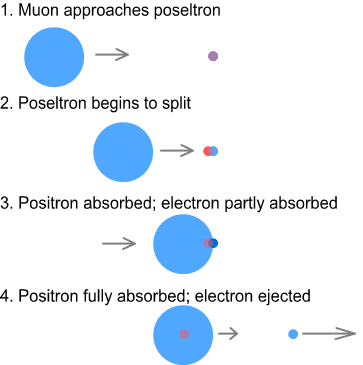The electron and positron are pictured smaller than the muon because they are lighter. If we assume sub-atomic particles to be made of a similar material of uniform density, this would make the muon/electron diameter ratio proportional to the cube-root of their mass ratio: in this case making the muon about 6 times larger as shown.

The idea that there should be direct correlation between mass and size seems quite logical and this is probably how most would view sub-atomic particles. But this view alas creates a problem for the electron-positron separation shown above. If the electron was much smaller than the muon its charge density would be much higher. Hence the muon would be unable to force the electron and positron to separate because the electron would be using a much higher percentage of its charge to attract and hold the positron. The only way the above could work is for the muon and electron to be very similar in size.

Based on this reasoning I’d like to make a bold hypothesis:

All fundamental (indivisible) sub-atomic particles are identical in size. They vary only in mass and charge.

If this is true, everything from an electron to a top quark has the same diameter but different densities. This may turn out to be true only for certain types of particles such as those in the  pion/muon/electron interactions. But if this principle can be extended to all particles, it would allow for a much broader range of interactions. Hence many composite particles could be composed from a small number of fundamental particles.

#### Conclusions

The conversion of cosmic protons into electrons, via pions and muons, can be understood in terms of particle absorption. In this manner no decay or creation of matter is involved and mass is preserved.

The existence of neutrinos is not required to explain particle interactions. If such particles do exist, they would have no effect on matter and be undetectable.

Protons are breakable. The up and down quarks that make up a proton are in fact positive pions and negative muons respectively. Quarks, like other particles, have unit charges; not fractional ones.

It’s possible that all fundamental particles are identical in size.

 http://en.wikipedia.org/wiki/Muon
 http://www2.slac.stanford.edu/vvc/cosmicrays/cratmos.html
 http://en.wikipedia.org/wiki/File:First_neutrino_observation.jpg

 Previous Contents Next chapter